# Historical Volatility Calculation

This page explains how to calculate historical volatility. If you are using Excel, see How to Calculate Historical Volatility in Excel or a ready-made Historical Volatility Excel Calculator.

## Introduction

While historical volatility is a well-known concept in finance, there is confusion in how exactly it is calculated. Different sources may use slightly different historical volatility formulas, so you can get different values for the same asset with the same settings in different software.

The following is the most common approach: calculating historical volatility as standard deviation of logarithmic returns, based on daily closing prices.

## What Historical Volatility Is Mathematically

When talking about historical volatility of securities or security prices, we actually mean historical volatility of returns. This may look like a negligible distinction, but it is very important for the calculation and interpretation of historical volatility.

Mathematically, historical volatility is the (usually annualized) standard deviation of returns.

If you know how to calculate return in a particular period and how to calculate standard deviation, you already know how to calculate historical volatility. Detailed step-by-step guide follows.

## Step 1: Deciding the Parameters

There are three parameters we need to set:

• The basic period (for which we calculate returns in the beginning) – often 1 day is used
• How many periods enter the calculation (we will refer to this as n) – often 20 or 21 days (the number of trading days and therefore the number of basic periods in one month)
• How many periods there are in a year (T; this is used for annualizing volatility in the end)

Personally, I mostly use 1 day (day-to-day returns), 21 or 63 days (representing 1 month or 3 months), and 252 (as there are 252 trading days per year on average).

It is not that important whether you use 20 or 21 days, or 252 or 262 days. It is much more important to use the same parameters consistently, so your results are comparable.

## Step 2: Calculating Logarithmic Returns

The actual calculation starts with calculating the continuously compounded return for each period. In our case, we will calculate the day-to-day returns for each of the 21 days (n = 21):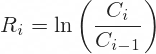... where:

ln = natural log
Cn = closing price
Cn – 1 = previous day closing price

## Step 3: Standard Deviation of Returns

Next we need to calculate standard deviation of the returns we got in the previous step.

Standard deviation is the square root of variance, which is the average squared deviation from the mean (see a detailed explanation of variance and standard deviation calculation).

First, calculate the average of the returns we got in step 2: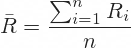Then, calculate the squared deviation from the average for each of the returns: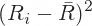Calculate the average of the squared deviations by summing them up and dividing by n – 1 (in our case 21 – 1 = 20). We are dividing by n – 1 rather than n, as we are calculating sample standard deviation (we are estimating the standard deviation from a sample – see the difference between population and sample standard deviation).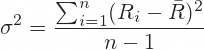We have just calculated variance of returns (σ2). Because volatility is standard deviation, not variance, we need to put the entire formula inside a square root:The number we got now (σ) is 1-day historical volatility (sample standard deviation of n daily logarithmic returns).

## Step 4: Annualizing Historical Volatility

The only thing left is to annualize the volatility: convert 1-day volatility to 1-year volatility, because that is the way it is typically quoted.

We do that by multiplying 1-day volatility by the square root of the number of (trading) days in a year – in our case square root of 252, which is approximately 16.

The result is annualized historical volatility.

## Historical Volatility Formula

The complete annualized historical volatility formula is: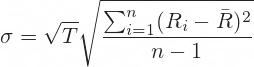... which is the same as: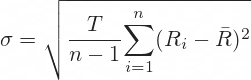... where:

n = historical volatility period
T = number of periods per year (number of trading days when calculating historical volatility from daily closing prices).

## Calculating Historical Volatility in Excel

In practice, calculating historical volatility manually would be lengthy and prone to errors. But it is very easy in Excel. In fact, the entire step 3 above can be done with the standard deviation Excel function (use STDEV.S for sample standard deviation).

## Historical Volatility Calculator

You can download the Historical Volatility Excel Calculator from Macroption. Besides the most popular HV calculation method described above, the calculator can also calculate HV using two other, alternative methods, including the zero mean (or non-centered) method. There is a user guide that comes with the calculator, which explains all the calculations in more detail.

Get the Historical Volatility Calculator + Guide now

By remaining on this website or using its content, you confirm that you have read and agree with the Terms of Use Agreement.

We are not liable for any damages resulting from using this website. Any information may be inaccurate or incomplete. See full Limitation of Liability.

Content may include affiliate links, which means we may earn commission if you buy on the linked website. See full Affiliate and Referral Disclosure.

We use cookies and similar technology to improve user experience and analyze traffic. See full Cookie Policy.